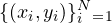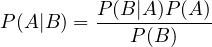In the previous articles, we had a short introduction to artificial intelligence and machine learning. In this article, we start to introduce the first technique for machine learning, Supervised Learning.

### What is Supervised Learning

If we read Wikipedia we can read this definition of Supervised Learning:

Supervised learning (SL) is the machine learning task of learning a function that maps an input to an output based on example input-output pairs. It infers a function from labeled training data consisting of a set of training examples. In supervised learning, each example is a pair consisting of an input object (typically a vector) and a desired output value (also called the supervisory signal).

However, for a better understanding of how a supervised algorithm works, let’s define some basic terms useful to build our algorithms.

How we know the fuel of every machine learning algorithm, is the data, a supervised learning algorithm used as an input a dataset as a collection of labelled data

More formally we can describe the dataset of the labelled data in this way:Each element of xi among N is called a feature vector. A feature vector is a vector in which each dimension, j=1,…., N contains a value that describes the example in analysis. This value is called feature and is denoted by the syntax x (j).

The goal of supervised learning is to predict the data in input based on the feature present on the data and then classify the data itself. Figure 1 shows how a feature work, in the figure we see the labelled data, Dog and the different weight, for example, 18 lbs or 14 lbs. A feature is essentially the characteristic connected with the data, the feature is important when we want to classify.

### Classification vs Regression

When we talk of Supervised Learning, the idea is generally to solve two major types of problems:

• Classification
• Regression

A classification problem is a type of problem where machine learning tries to classify the data based on the previous observation.

A typical example is a spam filter, the filter learns the common rules to classify the mail as spam or not, this type of classification is called binary classification.

Another type of classification is called multiclass classification, this type of classification can be used for example to recognize different types of animals, we feed the algorithm with different pictures and the algorithm classify the animal on unseen data.

The essence of the Classification is essential to assign a categorical label to unordered and unseen data.

There is another important task connected with Supervised Learning, this is called regression.

Regression analysis is used to predict a continuous outcome variable based on a prediction, if we want to be more formal, a regression analysis is for finding a correlation between some independent variable and one dependent variable. A classical example of regression analysis is the prediction of the price of a house based for example on the square feet or the location.

## Algorithm for Supervised Learning

We now have an idea about supervised learning, and what type of problem we can solve with this technique, for a better understanding of how to use let’s see the major algorithm used with supervised learning.

### K-Nearest Neighbor

The KNN or K-Nearest Neighbor is a simples algorithm used to classify data based on the similarity of the data. In the algorithm, the ‘k’ is used to identify the value of neighbour near the data point, this is used to analyze the value near the data point and then classify the data, new data are classified by the majority of vote of the neighbour, the number of neighbours used is identified by the value k.

### Naive Bayes

Naive Bayes classifier is a probabilistic algorithm, this is based on the Bayes Theorem and is used to solve a classification problem. This type of algorithm is essentially a probabilistic classifier, this means the classification is done using a probability of an event using the Bayes Theorem:Where:

• P(A|B) is the posterior probability, the probability of hypothesis A on the observed event B
• P(B|A) is the likelihood probability, the probability of an hypothesis is true
• P(A) is a prior probability, the probability of the hypothesis before observing the evidence
• P(B) is the marginal probability, the probability of the evidence

Naive Bayes is used for spam classification or text classification.

### Decision Trees

Decision Trees are used in both the problem from the supervised learning, Classification and Regression, for this reason, are sometimes called Classification And Regression Trees (CART).

In the Decision Trees, the prediction of the response is made directly by learning the feature derived from the features themselves.

In decision analysis, the decision tree can be used to visualize the data and explicitly represent decision and decision making.

### Linear Regression

Linear Regression is one of the most basic algorithms for Machine Learning, with the linear regression algorithm, the model tries to find the best linear between dependent and independent variables.

Linear regression can be split into two main types:

• Simple Linear Regression, where we have only one independent variable
• Multiple Linear Regression, where we have more than one independent variable

In both cases, the model tries to find the correlation between the independent variable and the dependent one.

### Support Vector Machine (SVM)

Support Vector Machine is another algorithm that can be used in both cases, classification and regression.

The SVM algorithm plot each data item as a point in the n-dimensional space, where n is the number of features we have in the model, each point represent a coordinate in the space.

With the coordinate defined, we use classification to find a hyper-plane used to differentiate the data.

## Conclusion

In this article, we introduce the supervised learning algorithm, this is the first algorithm used in Artificial Intelligence and Machine Learning.

In the next articles, we start to see how to implement some of the algorithms presented and we see how this algorithm can be used to build our Machine Learning model.

If you are interested to go deeper into the algorithm I can suggest some amazing books:

If you like the article feel free to add a comment and ask any question you can have